Technical Information Site of Power Supply Design

• Basic Knowledge
• AC/DC
• Design Example of Isolated Quasi-Resonant Converters Using SiC MOSFET
• Transformer T1 Design - 2

2018.09.06 AC/DC

# Transformer T1 Design - 2

Design Example of Isolated Quasi-Resonant Converters Using SiC MOSFET

In the previous section, “Transformer T1 Design – 1”, we explained the calculation procedure ① through ③ below. As Part 2 of this discussion, we here perform the remaining calculations ④ to ⑥, to complete design of the transformer T1.

• ①Setting a flyback voltage VOR
• ②Calculating the primary winding inductance Lp and
primary-side peak current Ippk
• ③Determining the transformer size
• ④Calculating the primary winding turns Np
• ⑤Calculating the secondary winding turns Ns
• ⑥Calculating the VCC winding turns Nd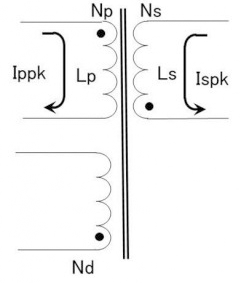As explained in the previous section, parameters that must be derived for the transformer design are the core size, the inductance Lp, and the winding turns Np, Ns, Nd. In the previous section, we already calculated the core size and the inductance Lp.

Parameters Necessary for Transformer Design

Transformer core size EFD30 or compatible 1750µH (to be calculated in ④) (to be calculated in ⑤) (to be calculated in ⑥)

In addition, conditions imposed on the transformer T1 are an output of 24 V at 1 A, and VIN(DC) of from 300 V to 900 V.

④Calculating the primary winding turns Np

As the fourth procedure, the number of primary winding turns Np is calculated. The maximum value of the flux density B(T) for general ferrite cores is 0.4 T at 100°C, and so we set Bsat = 0.3 T.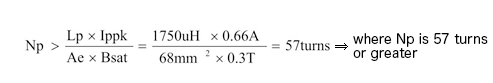In order to avoid magnetic saturation, the AL value-NI characteristic must be confirmed and care taken to ensure that the transformer is used in a region where saturation does not occur. For this purpose, we use an AL value-NI characteristic graph.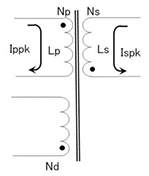For example, if we try setting Np = 50 turns, then we have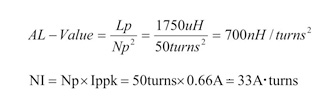and the saturation region is entered.
The number of primary winding turns is set so as to avoid the saturation region.
In the case of Np ＝ 64 turns,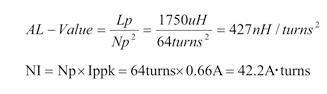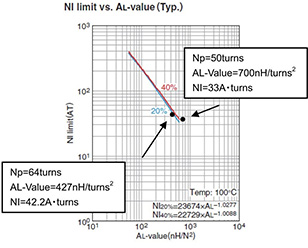and operation is in a region without saturation. Hence we set Np = 64 turns.

⑤Calculating the secondary winding turns Ns

Next, we calculate the secondary winding turns Ns. In “①Setting a flyback voltage VOR”, it was found that Np/Ns = 8, and so this is used here.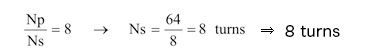⑥Calculating the VCC winding turns Nd

From the following equation, the number of VCC winding turns Nd is found. It is assumed that VCC = 24 V and Vf_vcc = 1 V.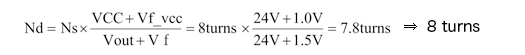The 24 V for VCC is the standard required voltage for the BD7682FJ-LB, which is the IC used in this design. A SiC MOSFET is being driven, and so a gate voltage (OUT pin clamp voltage) of 18 V (typ) is needed.

With this, calculation of all the required parameters is complete. We have inserted the numerical values into the table that appeared previously.

Parameters Necessary for Transformer Design

Transformer core size EFD30 or compatible 1750 µH 64 turns 8 turns 8 turns

To conclude, we present a design example for a transformer based on these parameters.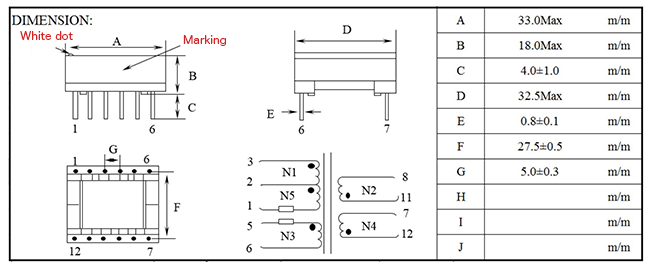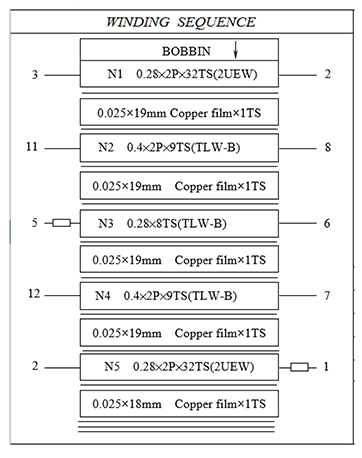#### Key Points:

・The core size, primary inductance and number of turns for a transformer T1 are calculated according to the procedure described.

・Calculations can be performed according to more or less the same approach as in “Designing Isolated Flyback Converter Circuits: Transformer Design (Calculating Numerical Values)”